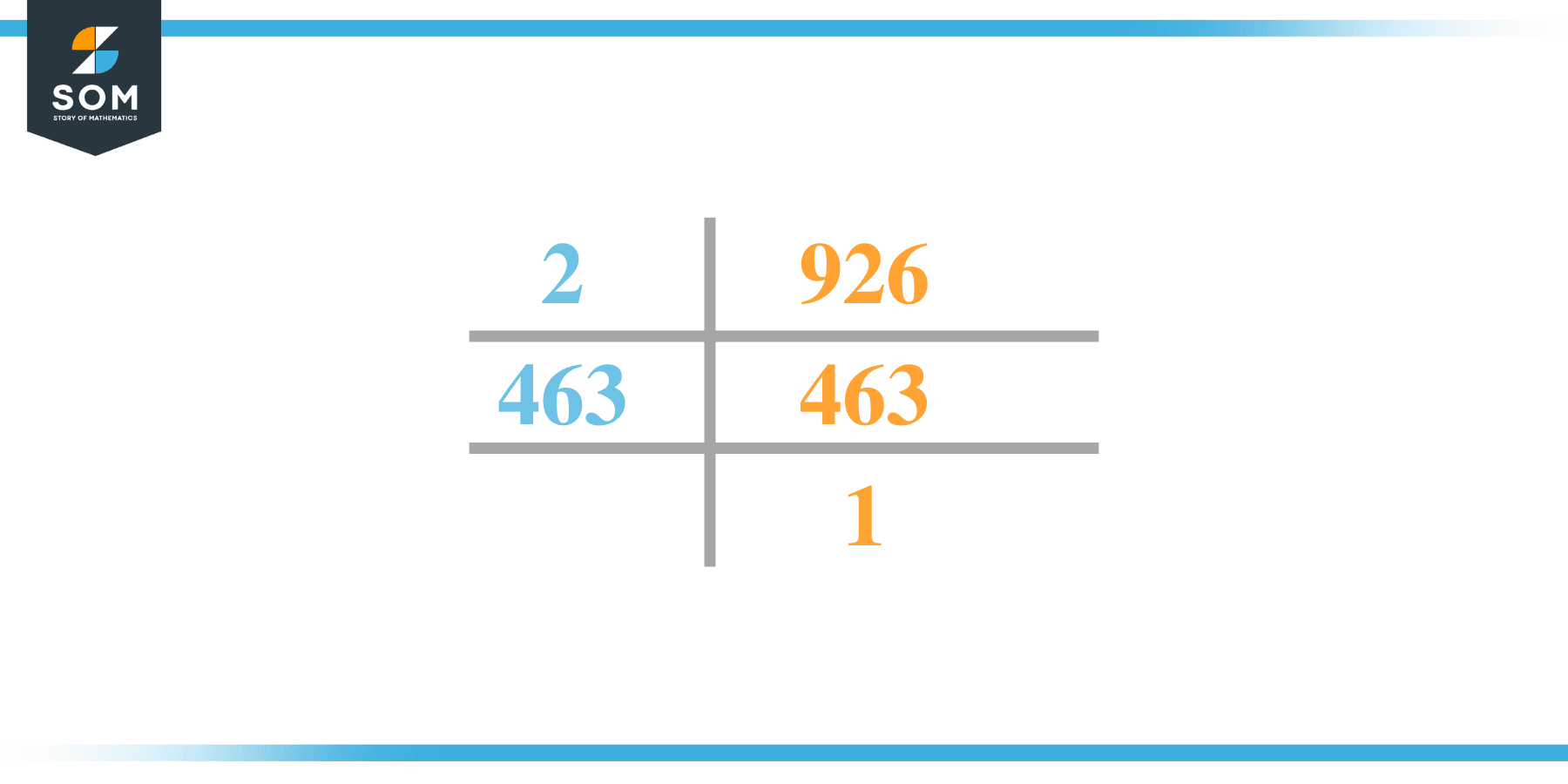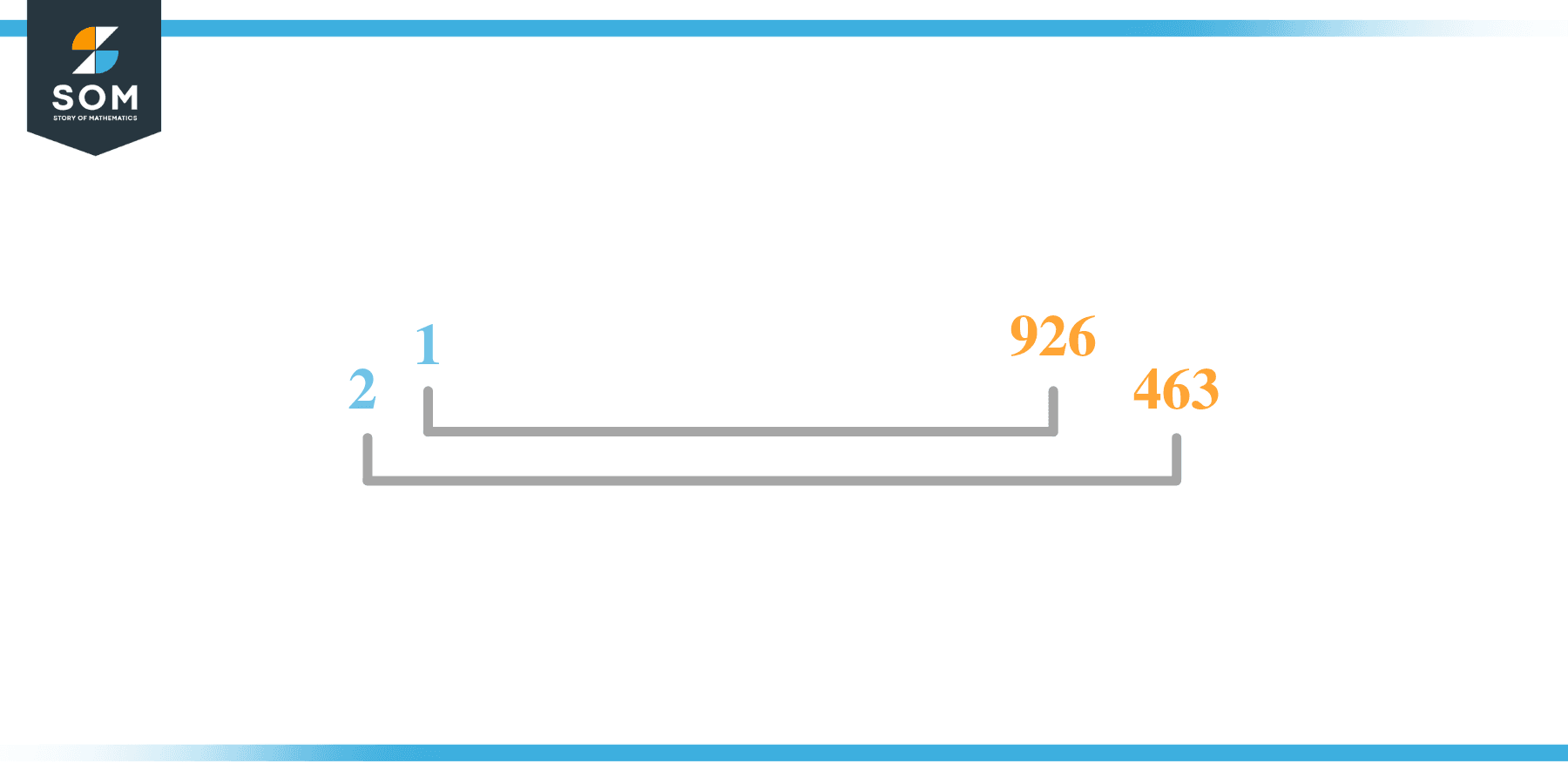# Factors of 926: Prime Factorization, Methods, and Examples

The number 926 is a multiple of the number 2 i.e. 2 x 463 =926. So as it divides 929 into two separate whole numbers 2 and 463 so we say that 926 is an even number but also if we divide these numbers 1, 2, 463, and 926 with 926 leaving 0 as the remainder so we said 926 is a composite with more than 2 factors.### Factors of 926

Here are the factors of number 926.

Factors of 926: 1, 2, 463, and 926

### Negative Factors of 926

The negative factors of 926 are similar to its positive aspects, just with a negative sign.

Negative Factors of 926: -1, -2, -463, and -926

### Prime Factorization of 926

The prime factorization of 926 is the way of expressing its prime factors in the product form.

Prime Factorization: 2 x 463

In this article, we will learn about the factors of 926 and how to find them using various techniques such as upside-down division, prime factorization, and factor tree.

## What Are the Factors of 926?

The factors of 926 are 1, 2, 463, and 926. These numbers are the factors as they do not leave any remainder when divided by 926.

The factors of 926 are classified as prime numbers and composite numbers. The prime factors of the number 926 can be determined using the prime factorization technique.

## How To Find the Factors of 926?

You can find the factors of 926 by using the rules of divisibility. The divisibility rule states that any number, when divided by any other natural number, is said to be divisible by the number if the quotient is the whole number and the resulting remainder is zero.

To find the factors of 926, create a list containing the numbers that are exactly divisible by 926 with zero remainders. One important thing to note is that 1 and 926 are the 926’s factors as every natural number has 1 and the number itself as its factor.

1 is also called the universal factor of every number. The factors of 926 are determined as follows:

$\dfrac{926}{1} = 926$

$\dfrac{926}{2} = 463$

$\dfrac{926}{463} = 2$

$\dfrac{926}{926} = 1$

Therefore, 1, 2, 463, and 926 are the factors of 926.

### Total Number of Factors of 926

For 926, there are four positive factors and four negative ones. So in total, there are eight factors of 926.

To find the total number of factors of the given number, follow the procedure mentioned below:

1. Find the factorization/prime factorization of the given number.
2. Demonstrate the prime factorization of the number in the form of exponent form.
3. Add 1 to each of the exponents of the prime factor.
4. Now, multiply the resulting exponents together. This obtained product is equivalent to the total number of factors of the given number.

By following this procedure, the total number of factors of 926 is given as:

Factorization of 926 is 2 x 463.

The exponent of 2 and 463 is 1, 1.

Adding 1 to each and multiplying them together results in four.

Therefore, the total number of factors of 926 is eight. Four are positive, and four factors are negative.

### Important Notes

Here are some essential points that must be considered while finding the factors of any given number:

• The factor of any given number must be a whole number.
• The factors of the number cannot be in the form of decimals or fractions.
• Factors can be positive as well as negative.
• Negative factors are the additive inverse of the positive factors of a given number.
• The factor of a number cannot be greater than that number.
• Every even number has 2 as its prime factor, the smallest prime factor.

## Factors of 926 by Prime FactorizationThe number 926 is a composite. Prime factorization is a valuable technique for finding the number’s prime factors and expressing the number as the product of its prime factors.

Before finding the factors of 926 using prime factorization, let us find out what prime factors are. Prime factors are the factors of any given number that are only divisible by 1 and themselves.

To start the prime factorization of 926, start dividing by its most minor prime factor. First, determine that the given number is either even or odd. If it is an even number, then 2 will be the smallest prime factor.

Continue splitting the quotient obtained until 1 is received as the quotient. The prime factorization of 926 can be expressed as:

926 = 2 x 463

## Factors of 926 in PairsThe factor pairs are the duplet of numbers that, when multiplied together, result in the factorized number. Factor pairs can be more than one depending on the total number of factors given.

For 926, the factor pairs can be found as:

1 x 926 = 926

2 x 463 = 926

The possible factor pairs of 926 are given as (1, 926) and (2, 463).

All these numbers in pairs, when multiplied, give 926 as the product.

The negative factor pairs of 926 are given as:

-1 x -926 = 926

-2 x -463 = 926

It is important to note that in negative factor pairs, the minus sign has been multiplied by the minus sign, due to which the resulting product is the original positive number. Therefore, -1, -2, -463, and -926 are called negative factors of 926.

The list of all the factors of 926, including positive as well as negative numbers, is given below.

Factor list of 926: 1, -1, 2, -2, 463, -463, 926, and -926

## Factors of 926 Solved Examples

To better understand the concept of factors, let’s solve some examples.

### Example 1

How many factors of 926 are there?

### Solution

The total number of Factors of 926 is four.

Factors of 926 are 1, 2, 463, and 926.

### Example 2

Find the factors of 926 using prime factorization.

### Solution

The prime factorization of 926 is given as:

926 $\div$ 2 = 463

463 $\div$ 463 = 1

So the prime factorization of 926 can be written as:

2 x 463 = 926